## The Digital Domain: Logic Gates

MF: lightly clean up to make the text more concise

Do we have any interest in salvaging the logic gates/XOR project that Selim and I worked on? --MF, 3/25/19

On this page, you review three Boolean operators (`and`, `or`, and `not`) and learn how they relate to electronic circuitry.

In a circuit with billions of transistors, or even thousands of transistors, hardware designers can't think about each individual transistor. Just as programmers use abstraction, hardware architects use abstractions, in which a group of transistors and other circuit elements are considered as a single thing. What kinds of things? There are basically two kinds, one for memory and one for computation.

Memory is made out of flip-flops. A flip-flop is a circuit that has two stable states, on and off. An input signal can tell it to turn on, turn off, or change whatever it's state is. Once that happens, the flip-flop stays in the new state until it gets another signal. It has an output that reflects its state: on if the flip-flop is on, off if it's off.

### Boolean Operators

The circuits to do computation are more interesting. They compute functions, just like reporters in Snap!. Since computers do a lot of arithmetic, you might think that the basic circuit functions would be addition, subtraction, multiplication, and division, but that's not the case. Of course there are circuits to do those things, but they're made out of logic gates: circuits that compute Boolean functions: `and`, `or`, and `not`.

The reason that Boolean functions are considered more fundamental is that their inputs and outputs can be represented with a single wire going into or out from the circuit. That's not true about arithmetic functions. If you consider a voltage on a wire as meaning 1, and no voltage as meaning 0, then you have to see that an adder will have three possible output values, because 1+1=2, which is neither 0 nor 1. By contrast, if you consider a voltage on a wire as meaning True, and no voltage as meaning False, then the output from a Boolean function of two inputs can still only be True or False, so only one output wire is needed.

You saw some examples in Unit 2 Lab 3: Making Decisions by Using Predicates: `AND`, `OR`, and `NOT`: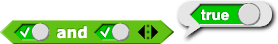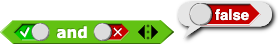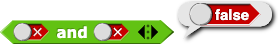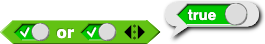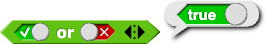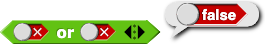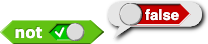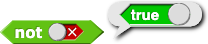Some other ways to think about Boolean operators: It is possible to emulate Boolean operations electronically and these operations are sometimes represented as truth tables (either with true/false or ones/zeros).
• A circuit emulation and truth tables for `and`.

This electric circuit shows a simplified way to emulate the Boolean operator `and` electronically. The two switches are in series; if both of the switches are on, the current flows and the light bulb turns on.

I wonder about making this simpler by including a pic of a battery in the animation. Students have a lot to manage in this endnote without this extra piece of information that isn't critical for the learning they are supposed to be doing. --MF, 12/4/17

This symbol in the circuit diagrams represents a battery.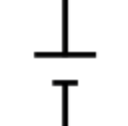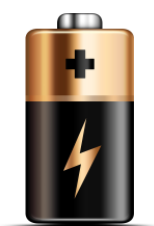These two tables are identical. One just shows the same pattern with true/false and the other with ones/zeros.
inputs output
A B A `and` B
False False False
False True False
True False False
True True True
inputs output
A B A `and` B
0 0 0
0 1 0
1 0 0
1 1 1
• A circuit emulation and truth tables for `or`.

This electric circuit shows a simplified way to emulate the Boolean operator `or` electronically. The two switches are in parallel; if either (or both) of the switches are on, the current flows and the light bulb turns on.

As with the `and` tables, these two tables are identical except for whether they use true/false or ones/zeros.

In ordinary language, the word "or" can have two slightly different meanings. Inclusive or means "at least one of these": If it's raining or it's really cold out, you need your overcoat. (If it's both raining and cold, you'd still wear the coat.) Exclusive or means "this or that, but not both": Eat your vegetables or you won't get any dessert. (You'd feel cheated if you ate the vegetables and still didn't get dessert.) In computer science (as in mathematics) the word "or" by itself always means inclusive or, as you can see in this truth table. If you mean "exclusive or," you have to say that.

inputs output
A B A `or` B
False False False
False True True
True False True
True True True
inputs output
A B A `or` B
0 0 0
0 1 1
1 0 1
1 1 1
• A circuit emulation and truth tables for `not`.

This electric circuit shows a simplified way to emulate the Boolean operator `not` electronically. This switch is basically a circuit breaker: if the switch is on, the current flow is broken and the light bulb turns off; if the switch is off, the current flows directly to the light bulb and the light bulb turns on.

As with the other tables, these are identical for true/false vs. ones/zeros.
input output
A `not` A
False True
True False
input output
A `not` A
0 1
1 0

## Logic Gates

Inside a computer, Boolean operations are implemented in physical circuitry using logic gates. (A single gate implements one of the basic functions `and`, `or`, or `not`.) Logic circuits are often represented with drawings that help engineers see how the information will flow through a circuit. For example, the following diagram of a logic circuit with two gates represents the Boolean expression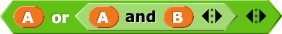. Can you see how?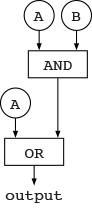1.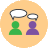Look at the logic circuit drawn above. For what values of A and B will the output be `true`?
On the previous page, you learned about tungsten and tin, and here we're talking about Boolean functions. In what sense is this less abstract? From the point of view of the chip designer, logic gates are the fundamental building blocks of a digital circuit. (The actual physical chip fabrication is at an even lower level of abstraction, in the analog domain.)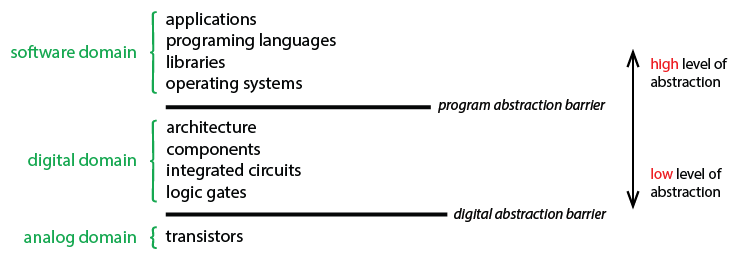1. Which of the following expressions will report `true`?
1.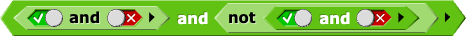2.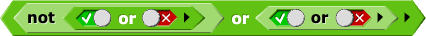I only
II only
I and II
Neither I nor II
2. Which of the following logic circuits will report `true`? (T stands for `true`, and F stands for `false`.)
This problem is probably harder than something you'll see on the exam, so if you can get this one, you are doing great!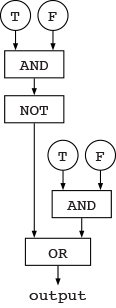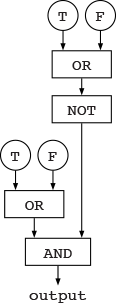I only
II only
I and II
Neither I nor II

How do engineers draw logic gates?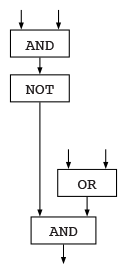Engineers typically draw logic gates horizontally and use special shapes that represent each gate: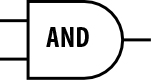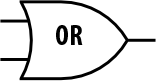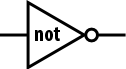For example, engineers would draw the logic circuit shown at right like this: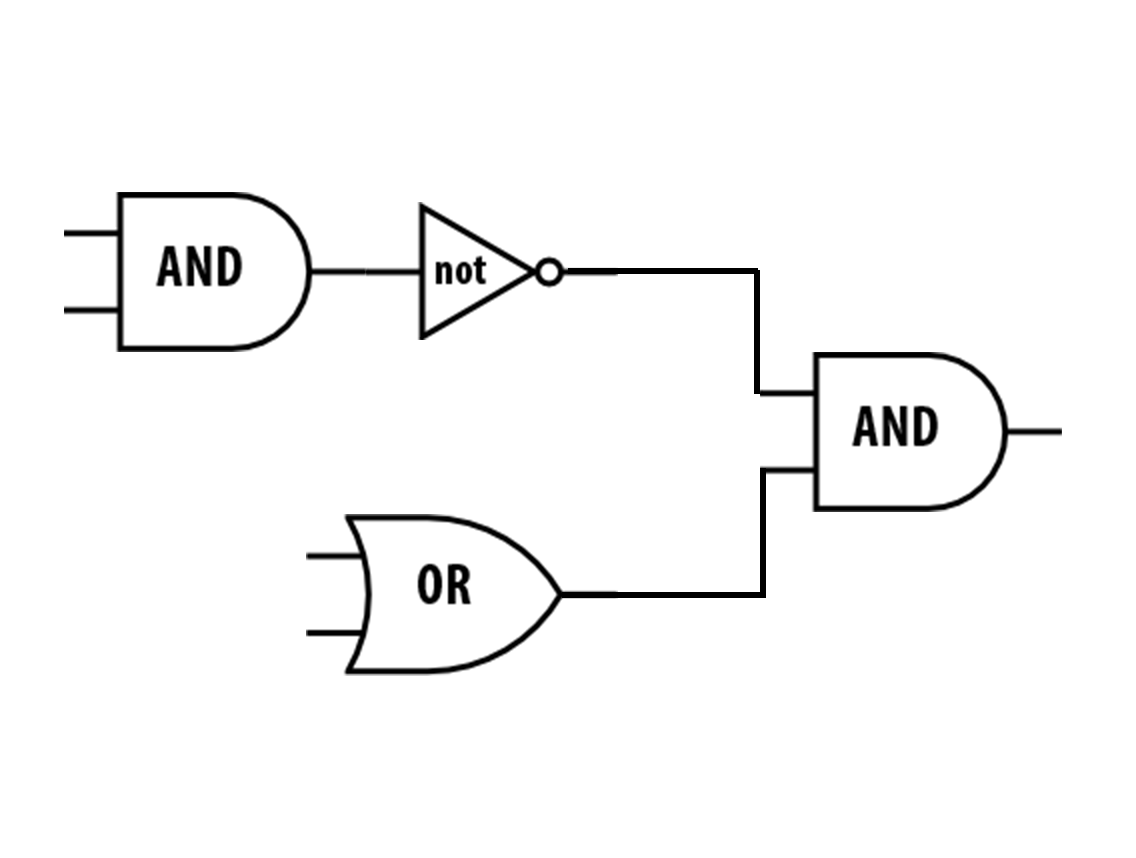Do an image search for "logic gate diagrams" to see more examples.
There is a commented out ifTime here that would link to the adder project. I think we should add it back in as a TIF once that optional project is done. --MF, 11/30/17